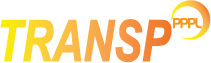# Boundary conditions for predictive runs

## Ion temperature predictions

MODIEDG=1
use the same boundary condition for the edge ion temperature as for the recycling neutrals at the edge. This is the default.
MODIEDG=2
use TIEDGE as a boundary condition. The deafult value is 10.0 eV.
Please note that, if this option is selected, the ion temperature is taken flat and equal to TIEDGE between XIBOUND and the separatrix.
MODIEDG=3
to use the edge electron temperature as boundary condition (from input data).
MODIEDG=4
to use the ion edge temperature as boundary condition (from input data).
MODIEDG=5
to use the pedestal width and height as boundary condition. Please refer to the pedestal module page for more detail.

## Recycling neutrals

If MODIEDG=1 the following settings also apply.

MOD0ED=1
use TIEDGE for the recycling neutral energy at the plasma boundary. This is the default.
MOD0ED=2
use TI0FRC*TI(center) for the recycling neutral energy at the edge. NOTE: this option is available only for particle balance calculations and not for predictive runs.
NOTE: need to set also TI0FRC, the multiplier of the central ion temperature.
MOD0ED=3
use edge electron temeprature data as boundary condition.
MOD0ED=4
use edge ion temeprature data as boundary condition.

## Electron temperature predictions

MODEEDG=2
use TEEDGE as boundary condition.
NOTE: if this option is selected, the electron temperature is taken flat and equal to TEEDGE between XIBOUND and the separatrix.
MODEEDG=3
use the edge electron temperature from input data as boundary condition. This is the default.
MODEEDG=5
to use the pedestal width and height as boundary condition. Please refer to the pedestal module page for more detail.

## Electron density predictions

MODNEDG=2
use EDGENE as boundary condition.
NOTE: if this option is selected, the electron density is taken flat and equal to EDGENE between XNBOUND and the separatrix.
MODNEDG=3
use the edge electron density from input data as boundary condition. This is the default.
MODNEDG=5
to use the pedestal width and height as boundary condition. Please refer to the pedestal module page for more detail.

## Rotation predictions

MODOMEDG=2
use OMEDGE as boundary condition.
NOTE: if this option is selected, the rotation profile is taken flat and equal to OMEDGE between XPHIBOUND and the separatrix.
MODNEDG=3
use the edge rotation from input data as boundary condition. This is the default.